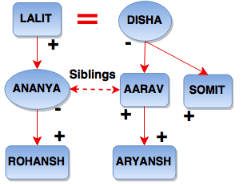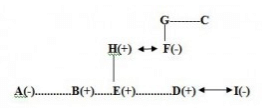# Blood Relation MCQ - 5

## 20 Questions MCQ Test Reasoning Aptitude for Competitive Examinations | Blood Relation MCQ - 5

Description
Attempt Blood Relation MCQ - 5 | 20 questions in 15 minutes | Mock test for Banking Exams preparation | Free important questions MCQ to study Reasoning Aptitude for Competitive Examinations for Banking Exams Exam | Download free PDF with solutions
QUESTION: 1

Solution:
QUESTION: 2

### Read the following information carefully and answer the question below.  1. Rohansh is the son of Aryansh’s father’s sister.  2. Somit is son of Disha who is mother of Aarav and Grandmother of Aryansh. 3. Lalit is the father of Ananya and grandfather of Rohansh.  4. Disha is wife of Lalit. How is Disha related to Rohansh?

Solution:

Disha is wife of Lalit, and Lalit is grandfather of Rohansh.
Rohansh is Disha’s grandson.QUESTION: 3

### A is sister of B. B is married to D. D is father of C. H is son of G. B is mother-in-law of G. D has only one son and no daughter. A is married to E. F is daughter of A. How is H related to D ? Q. How is A related to C ?

Solution:
QUESTION: 4

A is sister of B. B is married to D. D is father of C. H is son of G. B is mother-in-law of G. D has only one son and no daughter. A is married to E. F is daughter of A.
Q.

How  is B related to F ?

Solution:
QUESTION: 5

A is sister of B. B is married to D. D is father of C. H is son of G. B is mother-in-law of G. D has only one son and no daughter. A is married to E. F is daughter of A.
How is H related to D ?

Q.

K is the daughter of I. J is married to I. I is brother of L. H is married to K. How J related to H ?

Solution:
QUESTION: 6

If P+Q means P is the sister of Q, P-Q means P is brother of Q, P*Q means P is daughter of Q. Which of the following shows the relation that K is a uncle of O.

Solution:
QUESTION: 7

M and N are sisters. X and Y are brothers. M’s daughter is X’s sister. what is N’s relation to Y?

Solution:
QUESTION: 8

A is sister of B. E is brother of B. E is son of H. H is married to F. F is daughter of G. F has only one daughter. G is married to C. E is brother of D. D is married to I.
How is I related to A ?

Solution:QUESTION: 9

A is sister of B. E is brother of B. E is son of H. H is married to F. F is daughter of G. F has only one daughter. G is married to C. E is brother of D. D is married to I.
How is I related to A ?

Q.

If M is brother of C then how is G related to M ?

Solution:
QUESTION: 10

A is sister of B. E is brother of B. E is son of H. H is married to F. F is daughter of G. F has only one daughter. G is married to C. E is brother of D. D is married to I.
How is I related to A ?

Q.

If R is daughter of I then how B is related to R ?

Solution:
QUESTION: 11

A×B means A is brother of B
A÷B means A is sister of B
A+B means A is father of B
A-B means A is mother of B
Which of the following means P is nephew of K ?

Solution:
QUESTION: 12

A×B means A is brother of B
A÷B means A is sister of B
A+B means A is father of B
A-B means A is mother of B
Which of the following means F is grandfather of G ?

Solution:
QUESTION: 13

A×B means A is brother of B
A÷B means A is sister of B
A+B means A is father of B
A-B means A is mother of B
Which of the following means P is nephew of K ?

Solution:
QUESTION: 14

A×B means A is brother of B
A÷B means A is sister of B
A+B means A is father of B
A-B means A is mother of B
Micael said, pointing to Mahavir “He is son of my granfather’s daughter’”.How is Micael related to Mahavir ?

Solution:
QUESTION: 15

N means M is Sister of N
M@N means M is Father of N
M÷N means M is Son of N
M%N means M is Mother of N
What will come in the place of ?. If P is grandson of Q is to be true in the given expression  Q % T @ P ? S

Solution:
QUESTION: 16

N means M is Sister of N
M@N means M is Father of N
M÷N means M is Son of N
M%N means M is Mother of N
In which of the following pairs is the first person daughter of the second person with regard to the expression F @ G ÷ I + J ÷ H

Solution:
QUESTION: 17

Pointing to a lady in the photograph Kiran said, “She is the daughter of the daughter of the only son of my grand father.” How is the lady related to Kiran ?

Solution:
QUESTION: 18

Pointing to a lady in photograph, Nitesh said, “Her mother is the only daughter of my mother’s mother.” How is Nitesh related to the lady?

Solution:
QUESTION: 19

​‘P × Q’ means ‘P is son of Q’.
‘P + Q’ means ‘P is daughter of Q’.
‘P ÷ Q’ means ‘P is wife of Q’.
‘P – Q’ means ‘P is father of Q’.

Q.

In the expression ‘K + H – P ? Q’, what will come in place of ? if Q is mother of K?

Solution:
QUESTION: 20

‘P × Q’ means ‘P is son of Q’.
‘P + Q’ means ‘P is daughter of Q’.
‘P ÷ Q’ means ‘P is wife of Q’.
‘P – Q’ means ‘P is father of Q’.

Q.

Which of the following relation is true with regard to expression ‘B ÷ P × Z – K + O’?

Solution:Use Code STAYHOME200 and get INR 200 additional OFF Use Coupon Code